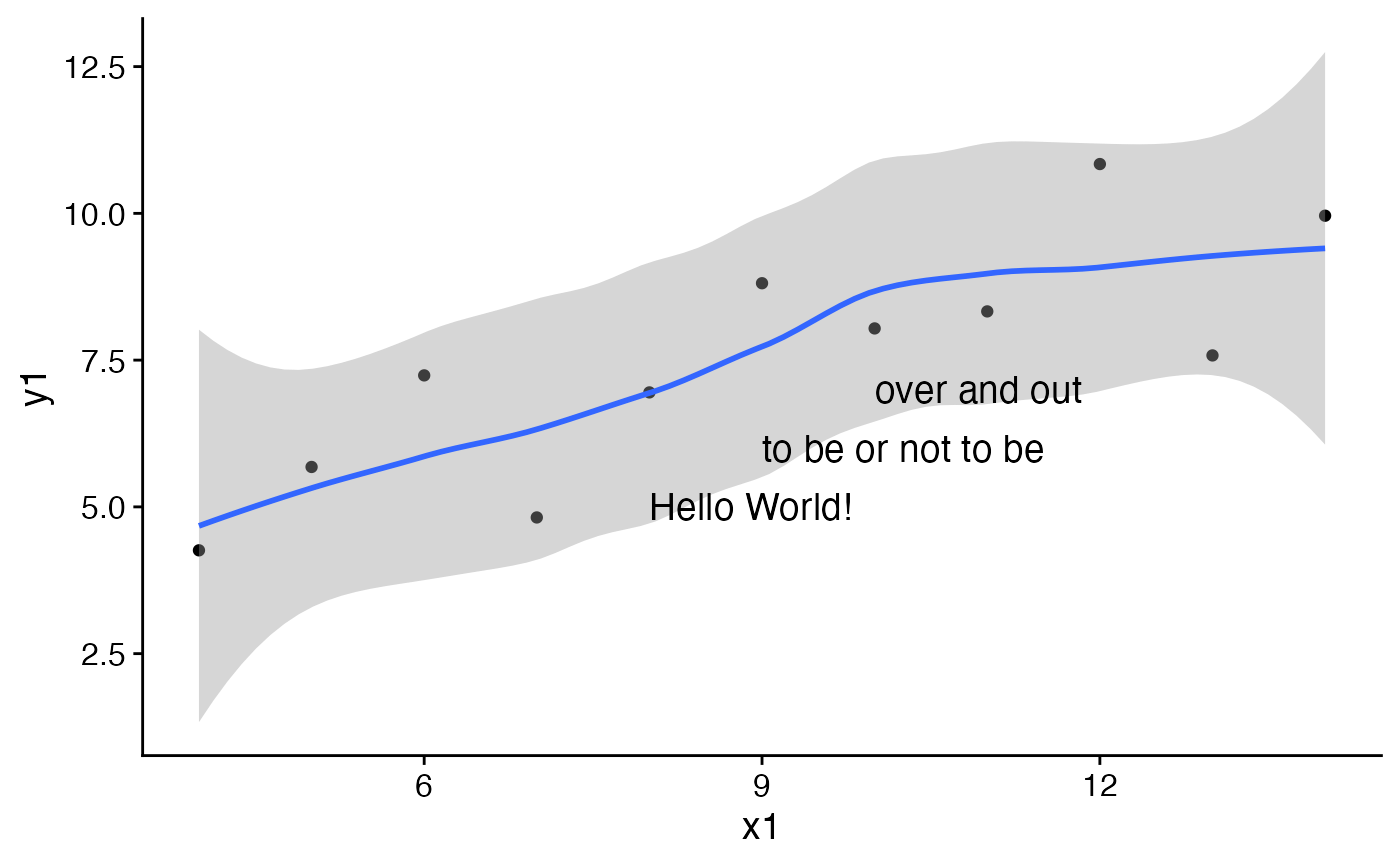This is a convenience function to plot multiple pieces of text at the same time. It cannot handle mathematical expressions, though. For those, use draw_label.

draw_text(text, x = 0.5, y = 0.5, size = 14, hjust = 0.5, vjust = 0.5, ...)

Arguments

text A vector of Character (not expressions) specifying the string(s) to be written. Vector of x coordinates. Vector of y coordinates. Font size of the text to be drawn. (default = 0.5) (default = 0.5) Style parameters, such as colour, alpha, angle, size, etc.

Details

Note that font sizes are scaled by a factor of 2.85, so sizes agree with those of the theme. This is different from geom_text in ggplot2.

By default, the x and y coordinates specify the center of the text box. Set hjust = 0, vjust = 0 to specify the lower left corner, and other values of hjust and vjust for any other relative location you want to specify.

For a full list of ... options, see geom_label.#> geom_smooth() using method = 'loess' and formula 'y ~ x'